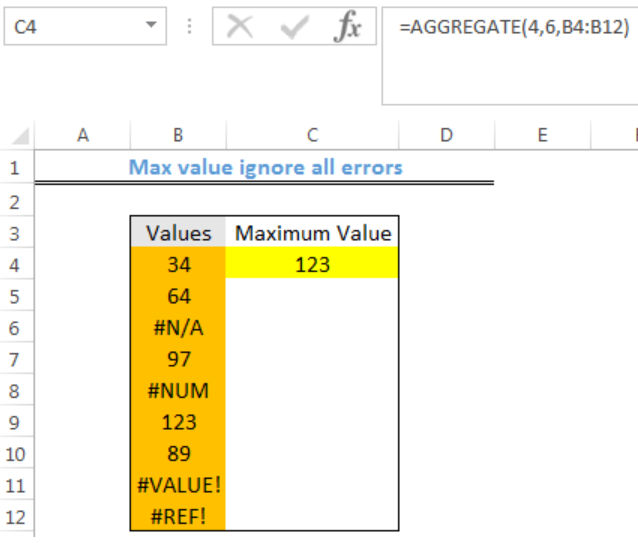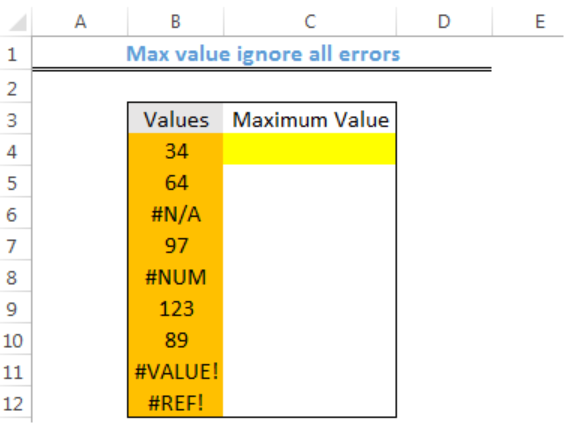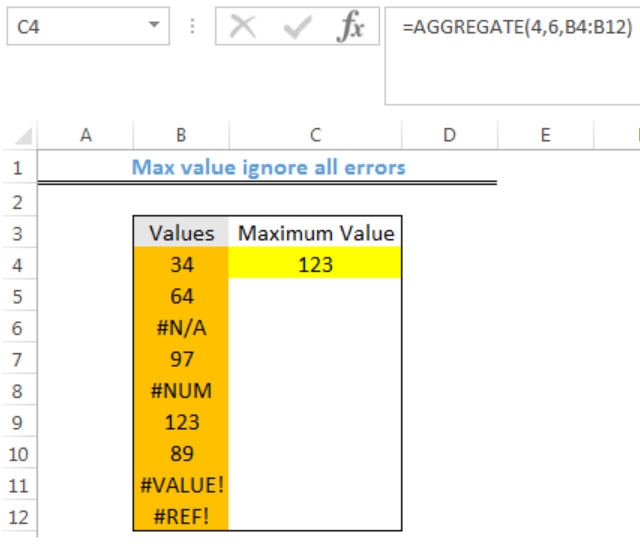Get instant live expert help with Excel or Google Sheets“My Excelchat expert helped me in less than 20 minutes, saving me what would have been 5 hours of work!”

#### Post your problem and you’ll get Expert help in seconds.

Your message must be at least 40 characters
Our professional Expert are available now. Your privacy is guaranteed.

# Max value ignore all errors

We can use the AGGREGATE function to calculate the maximum numerical value in a set of values full of errors. We can calculate the maximum value from the data using max value ignore all errors task through Excel Aggregate function. The steps below will walk through the process.Figure 1: Maximum Value obtained and All Errors Ignored

## General Formula

`=AGGREGATE(4,6,range of values)`

## Formula

`=AGGREGATE(4,6,B4:B12)`

## Setting up the Data

• We will set up data by inputting our Values in Column B
• The result will be returned in Cell C4Figure 2: Setting up the Data

## Get Maximum Value and Ignore All Errors

• We will click on Cell C4
• We will insert the formula below into the cell
`=AGGREGATE(4,6,B4:B12)`
• We will press the enter keyFigure 3: Maximum Value obtained and All Errors Ignored

## Explanation

The AGGREGATE function can ignore errors optionally when we are calculating the maximum value in a set of data. In the formula, the number 4 specifies average and the 6 serves as an option to ignore errors.

## Note

• MAXIFS is an alternative to Max Value Ignore All Errors
• If the data set value is positive, with the formula below, we can get the maximum  number and ignore all errors:

`=MAXIFS(values,values,">=0")`

In this formula, the “greater” or “equal to zero” expression removes error values and provides the maximum numerical value.

## Instant Connection to an Expert through our Excelchat Service

Most of the time, the problem you will need to solve will be more complex than a simple application of a formula or function. If you want to save hours of research and frustration, try our live Excelchat service! Our Excel Experts are available 24/7 to answer any Excel question you may have. We guarantee a connection within 30 seconds and a customized solution within 20 minutes.

### Did this post not answer your question? Get a solution from connecting with the expert.Another blog reader asked this question today on Excelchat:
Solution examplesI'm trying to put the search and output lists for the following formula on a separate sheet. But simply selecting the data on that sheet won't work. =IFERROR(INDEX(E\$2:E\$6, AGGREGATE(15, 6, ROW(\$1:\$6)*SIGN(MATCH("*"&D\$2:D\$6&"*", \$A2, 0)), 1)),"")
Solved by X. W. in 31 mins## Subscribe to Excelchat.coAnother blog reader asked this question today on Excelchat: